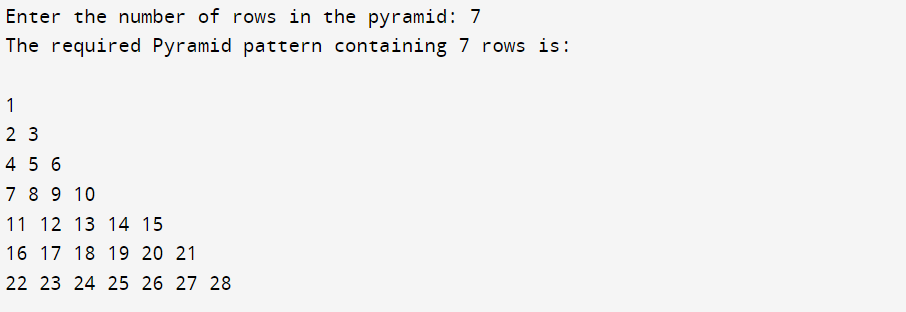top of page
Search

# Write a program to print Floyd's Triangle in C++.

### Floyd's Triangle

Floyd's triangle is a right-angled triangular array of natural numbers. It is defined by filling the rows of the triangle with consecutive numbers, starting with a 1 in the top left corner:

All such patterns using * or alphabets or numbers are achieved by making use of the nested loop structures by knowing how to iterate and till where to iterate.

Code:

```using namespace std;

int main()
{
//i to iterate the outer loop and j for the inner loop
int i, j, rows, cols;

//to denote the range of numbers in each row
int n=1, first,last;

cout << "Enter the number of rows in the pyramid: ";
cin >> rows;
cout << "\n\nThe required Pyramid pattern containing " << rows << " rows is:\n\n";

//outer loop is used to move to a particular row
for (i = 1; i <= rows; i++)
{

first = n;
last  = first + i -1;
cols = i;

//to display that the outer loop maintains the row number
//cout << "Row # " << i << " contains the numbers from " << first << " to " << last << " :    ";

//inner loop is used to decide the number of * in a particular row
for (j = 1; j<= cols; j++)
{
cout << n << " ";
n+=1;
}

cout << endl;
}

cout << "\n\n";
return 0;
}```

Output:The Tech Platform